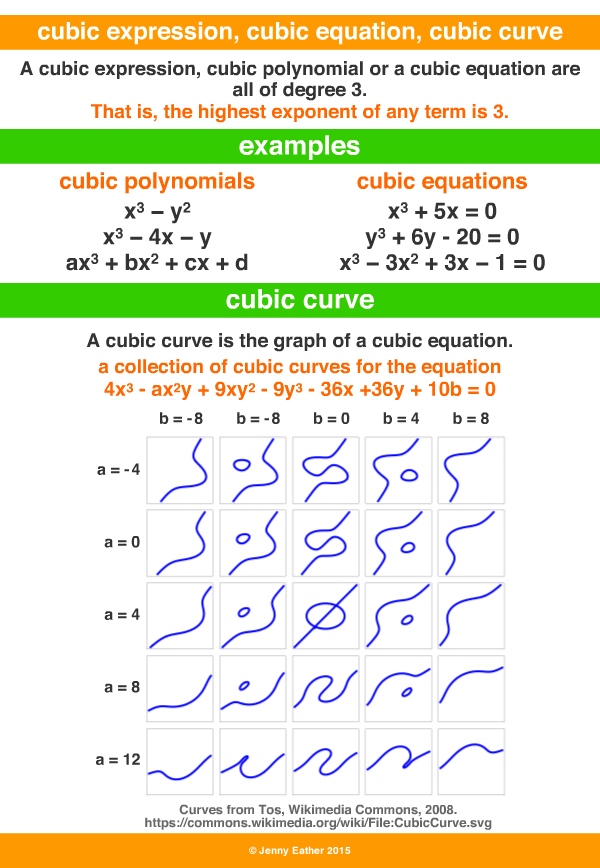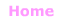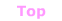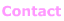Aa Bb Cc Dd Ee Ff Gg Hh Ii Jj Kk Ll Mm Nn Oo Pp Qq Rr Ss Tt Uu Vv Ww Xx Yy Zz

Cc

cubic expression, equation and curve

• an expression, polynomial or equation of degree 3,
degree 3 or to the power of 3 means cubed.
• a cubic curve is the graph of a cubic equation.

EXAMPLES:interactive cubic function explorer
http://www.mathopenref.com/cubicexplorer.html© Jenny Eather 2014. All rights reserved.